# Interactive Data Analysis with the Apache Spark Shell

Rating: 4

3029

Spark Shell For Interactive Analysis

With the Spark shell, we can easily learn on how to use the API, and a powerful tool for an interactive analysis of data will be provided. The shell for Spark is available in Python or Scala. Note that Scala runs on the Java Virtual Machine (JVM).

Are you intereted in taking up for Apache Spark Certification Training? Enroll for Free Demo on  Apache Spark Training!

Start the shell by navigating to the Spark directory, and then execute the following command:

For Scala users, execute the following command:For Python users, run the following command:The primary abstraction for Spark is the RDD (Resilient Distributed Dataset) which is a distributed collection of items. To create RDDs, we can transform other RDDs or create them from the Hadoop InputFormats.

The README file contained in the root directory of Hadoop has some text. Let us try to make an RDD from this text by executing the following command: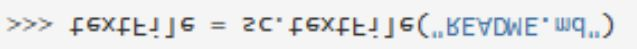With RDDs, there are actions which return values, and there are transformations which will return pointers to other RDDs. Consider the actions given below: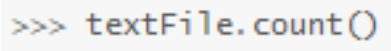The above command should give us the number of items which are contained in our RDD. Consider the next command given below: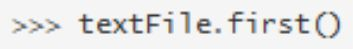The command given above will give us the first item which is contained in our RDD.

We now need to make use of a transformation. The filter transformation will be used for returning a new RDD having a subset of the items which are contained in the file. This is shown below: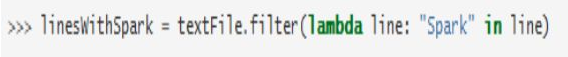The actions and the transformations can then be chained together as shown below: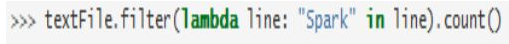The actions and transformations for RDD can be used for carrying out of more complex computations. Consider a scenario in which we need to find the line which is having the most words. This can be done by use of the command given below:

textFile.map(lambda line: len(line.split())).reduce(lambda x, y: x if (x > y) else y)

With the above line of code, the line will first be mapped to an integer value, and a new RDD will be created. The function “reduce” will then be called on the newly created RDD in that line, so that the largest line count is found. Consider the example given below:

def max(x, y):
… if x > y:
… return x
… else:
… return y

Once you have written the above, the following command should then be executed:

textFile.map(lambda line: len(line.split())).reduce(max)

MapReduce is one of the most command data flow patterns which are supported in Spark. This can easily be implemented in Spark as shown below:

wc = textFile.flatMap(lambda line: line.split()).map(lambda word: (word, 1)).reduceByKey(lambda x, y: x+y)

Note that in the above example, we have combined several transformations for the computation of the per word count in our file as an RDD of pairs of string and integer.

The “collect” action can be used for collection of the word count in the shell as shown below: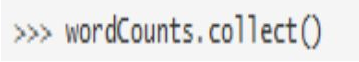Caching

With Spark, one can pull the data sets into a memory cache which is cluster-wide. This becomes very important in circumstances where the data has to be accessed repeatedly. A good example is when the algorithm that you are using is iterative. The data will not have to be fetched from the memory, which involves much overhead, but from the cache, which is faster and offers much less overhead. We need to demonstrate how caching can be done. Suppose that you want to mark a particular line to be cached, this can be done as follows: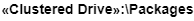Note that you do not have to do caching on files which have very few lines. It is recommended that this should be done on files which have large data sets. Even if the data sets have been distributed across multiple nodes, the functions can be applied on them. The process can also be done interactively.Writing Self-Contained Applications

Sometimes, you might need to use the Spark API so as to create self-contained applications. This can be done in Java, Scala, and Python.

The Python API, that is, PySpark, can be used for writing self contained applications.

Scala

We need to create a simple self contained app with Scala. The code given below can be used for that purpose:

```/* MyApp.scala */
import org.apache.spark.SparkContext._
import org.apache.spark.SparkContext
import org.apache.spark.SparkConf
object MyApp {
def main(args: Array[String]) {
val lFile = “YOUR_SPARK_HOME/README.md” // The file should be present in
val con = new SparkConf().setAppName(“My Application”)
val sc = new SparkContext(con)
val lData = sc.textFile(lFile, 2).cache()
val nAs = lData.filter(line => line.contains(“x”)).count()
val nBs = lData.filter(line => line.contains(“y”)).count()
println(“Lines with x: %s, Lines with y: %s”.format(nAs, nBs))
}
}```

With the above example, the number of lines containing the letter “x” and the ones containing the letter “y” will be counted. These will be counted in the README file. The parameter “YOUR_SPARK_HOME” in the above code should be replaced with the location of Spark in your local system, otherwise, you will get an error. You also notice that we have initialized our own SparkContext, unlike what we have doing in the other examples. A repository on which Spark will depend on will also be created as shown below:

```name := “My Project”
version := “1.0”
scalaVersion := “2.10.4”
lDependencies += “org.apache.spark” %% “spark-core” % “1.5.0”```

We must lay the app according to the typical structure of the directory. It is after this that a JAR package containing the code for the application can be created, and then we will execute or run the program.

Learn Apache Spark Tutorial

Java

The following code can be used for creation of a simple Spark application in the Java programming language:

```/* MyApp.java */
import org.apache.spark.SparkConf;
import org.apache.spark.api.java.*;
import org.apache.spark.api.java.function.Function;
public class MyApp {
public static void main(String[] args) {
String lFile = ” SPARK_HOME/README.md”; // This file should be available in
SparkConf con = new SparkConf().setAppName(“My Application”);
JavaSparkContext sc = new JavaSparkContext(con);
JavaRDD lData = sc.textFile(logFile).cache();
long nAs = lData.filter(new Function() {
public Boolean call(String s) { return s.contains(“x”); }
}).count();
long nBs = lData.filter(new Function() {
public Boolean call(String s) { return s.contains(“y”); }
}).count();
System.out.println(“Lines with x: ” + nAs + “, lines with y: ” + nBs);
}
}```

Similarly, the program given above will count the number of lines in the file README for the Spark which have the letters “x” and “y”. The parameter “SPARK_HOME” has to be replaced with the location of the Spark in your system, otherwise, the program will not run. Note that we have also initialized a SparkContext, unlike in the other cases.

For the purpose of building the application, a Maven “pon.xml” file should also be written and this will be used for listing Spark as a dependency. The artifacts for Spark are tagged with a version for Scala. This is shown below:

That is how it looks like.

Python

A simple Spark application can also be created by use of the Python API, that is, PyAPI. The following code can be used for creating the application “MyApp.py”:

```“””MyApp.py”””
from pyspark import SparkContext
lFile = ” SPARK_HOME/README.md” This file should be available in your local
system.
sc = SparkContext(“local”, “My App”)
lData = sc.textFile(logFile).cache()
nAs = logData.filter(lambda s: ‘x’ in s).count()
nBs = logData.filter(lambda s: ‘y’ in s).count()
print(“Lines with x: %i, lines with y: %i” % (nAs, nBs))```

The above program will be used for counting the number of lines having the letters “x” and “y” in the file README of the Spark. Again, do not forget to replace the parameter “SPARK_HOME” with the location of the Spark installed on your system.

Consider the code given below, which shows how a simple job can be implemented in Java:

```/*** MyJob.java ***/
import spark.api.java.*;
import spark.api.java.function.Function;
public class MyJob {
public static void main(String[] args) {
String lFile = “/var/log/syslog”; // The file should be available in your local system
JavaSparkContext sc = new JavaSparkContext(“local”, “My Job”,
“\$ SPARK_HOME”, new String[]{“target/my-project-1.0.jar”});
JavaRDD lData = sc.textFile(lFile).cache();
long nAs = lData.filter(new Function() {
public Boolean call(String s) { return s.contains(“x”); }
}).count();
long numBs = logData.filter(new Function() {
public Boolean call(String s) { return s.contains(“b”); }
}).count();
System.out.println(“Lines with x: ” + nAs + “, lines with y: ” + nBs);
}
}```Course Schedule
NameDates
Apache Spark TrainingJan 31 to Feb 15
Apache Spark TrainingFeb 04 to Feb 19
Apache Spark TrainingFeb 07 to Feb 22
Apache Spark TrainingFeb 11 to Feb 26
Last updated: 29 January 2023